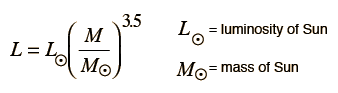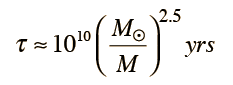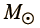The luminosity of a star is a measure of its energy output, and therefore a measure of how rapidly it is using up its fuel supply. The lifetime of a star would be simply proportional to the mass of fuel available divided by the luminosity if the luminosity were constant. Beyond these statements, one must rely on the empirical data collected and models of that data to estimate the lifetime of a given star.

One useful step toward modeling stellar lifetimes is the empirical mass-luminosity relationship.Since the mass of the star is the fuel for the nuclear fusion processes, one could then presume that the lifetime on the main sequence is proportional to the stellar mass divided by the luminosity. It depends upon the fraction of mass that is actually available as nuclear fuel, and considerable effort has gone into modeling that fraction for the Sun to yield a solar lifetime of 10 x 109 years. Using that projected lifetime, the stellar lifetime can then be expressed asFor a star of massThis model projects a lifetime of τ = x109 years

τ = million years.

Note that this is just a rough model and that the number of digits in the model calculation is certainly not significant. Only the first two digits should be considered to be significant. If we take into account the range of powers commonly associated with the power relationship, the uncertainty in the model lifetime can be appreciated.

For a power of 2 in the lifetime relationship, τ = x109 years

For a power of 3 in the lifetime relationship, τ = x109 years

Index

Star processes

Galaxy concepts

Reference
Harwit
Ch 8

 HyperPhysics***** Astrophysics R Nave
Go Back

Star Times

Star lifetimes are so vast compared to the time that we have been studying them that we must rely on modeling and the observation of stars at different stages of development to make estimates of stellar development times. Such modeling might break up the stages of development as

Index

Star processes

Galaxy concepts

Reference

 HyperPhysics***** Astrophysics R Nave
Go Back